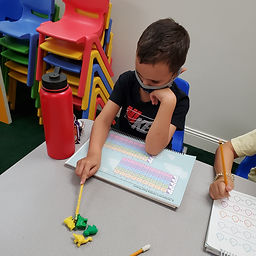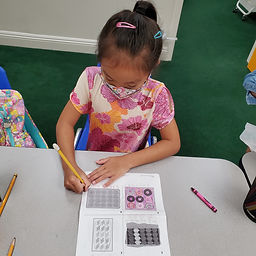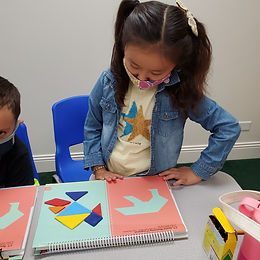## Ms. Raejean

### Target 1​

###### Lesson Type:

New

Number Operation

:

Computation

Work with equal groups of numbers to gain foundations for multiplication (repeated addition).

###### 2:

Set the foundation for later exploration of multiplication (i.e. 2+2+2+2 =? How many 2s did you add? When you add 4 number 2s together, what do you get?)

###### 3:

Use addition to find the total number of objects in an array.

2nd

###### Vocabulary:

Activities:

Students used repeated addition to count a group of objects. First, students circled equal groups of items. Then, students wrote an addition equation. Next, students found the sum of equal addends. This will help build the foundation for multiplication.### Home Exploration

###### Guiding Questions:## Absent Students:

### Target 2

:

###### 1:

Solve simple tangram puzzles by recognize which spaces in the puzzle the given seven shapes can and cannot fit.

2nd

###### Vocabulary:

Tangrams, Tans, Triangles, Square, Parallelogram

Activities:

Students learned about tangrams, a puzzle made up of 7 shapes (tans). Parallelogram was introduced. Students used visual and spatial reasoning to solve the Tangram puzzle. Students turned, flipped, and slid the tans to cover up each puzzle.### Home Exploration

###### Guiding Questions:### Target 3

:

###### Vocabulary:

Activities:### Home Exploration